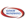# Activities

••• ##### Subject Area

• Math: Algebra I: Exponential Functions
• Math: Algebra I: Linear Functions
• Math: Algebra I: Quadratic Functions

• ##### Author6-8
9-12

30 Minutes

• ##### Device
• TI-83 Plus Family
• TI-84 Plus
• TI-84 Plus Silver Edition
• ##### Other Materials
For more information on the Glencoe/McGraw-Hill mathematics series visit them on the web at www.glencoe.com

## Glencoe Algebra 1: Graphing Calculator Investigation - Curve Fitting

#### Activity Overview

This Graphing Calculator Investigation activity is from Glencoe Algebra 1 Chapter 13: Statistics, Lesson 13-3: Histograms. Students will make scatter plots and find the appropriate regression equations that best fit the data.

#### Before the Activity

Review the activity PDF file.
Make copies of the activity PDF file for your class.

#### During the Activity

Students will:

• Make scatterplots
• Use the LinReg, QuadReg, and ExpReg functions of the graphing calculator to find the appropriate regression equations that best fits the data - linear, quadratic or exponential
• State the coefficient of determination

• Directions:
• Distribute the student activity sheets
• Follow the procedures as outlined
• #### After the Activity

As a class, review student answers, discussing questions that appeared to be more challenging, and re-teaching as needed.